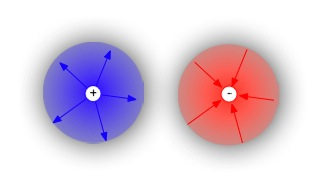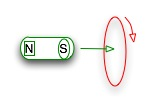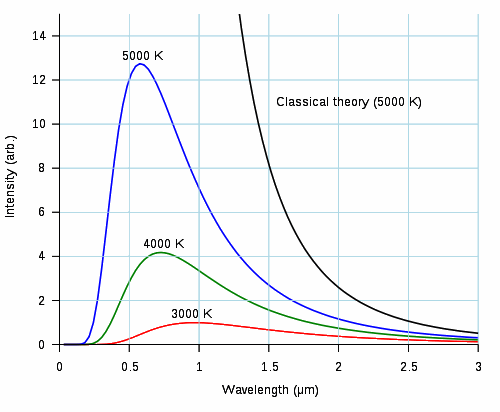# ChemistryWebLecture

## Light

A series of discoveries led Faraday and Maxwell to realize that electricity and magnetism were related and able to generate each other, and the self-propagating wave they generated was light.

Radiation is the transmission of energy across space — with or without a medium. Our modern theories of light are based on discoveries about electricity, magnetism, and the interaction of light and matter.

Earth in the seventeenth century, Galileo tried to determine th speed of light by sending a servant far out into the fields to open a lantern at a prearranged time. When he could detect no discernable difference in the arranged time and the arrival of the beam of light from the lantern, he concluded that the speed of light was very fast, possibly instantaneous.

In the late seventeenth century, the Danish astronomer Ole Roemer used Newton's laws to predict the orbital periods of Jupiter's moons. He determined that the observed deviations from the predicted times could be explained if light had a finite speed, and using the difference between prediction andobsrvation, calculated the speed of light. Modern estimates put this amount at just under 3 * 105 km/sec.

However, there remained the debate on the actual nature of light. Isaac Newton conceived of light as small particles that moved through space; different colors had different properties. However, Christiaan Huygens thought of it as a form of wave. Experimental evidence to support Huygen's theory was supplied by Thomas Young's double slit experiments, which showed that light going through a pair of narrow slits produces a diffraction pattern similar to that of waves in water passing through similar barriers.

You can create create this effect by looking with one eye at a light source (a room or table lamp is sufficient) through the slit produced by holding your fingers close together and close to your eye. You should be able to see one or more dark lines alongside the actual edges of your fingers. These are the minima (equivalent to still water) where light waves striking each edge of the slit are diffracted and interfere with one another.

During the eighteenth and nineteenth centuries, Grey, Franklin, Ampere, Gauss, and others observed that electrically charged particles could affect each other and magnetic materials in particular ways. First, they noticed that electrical charge could arise in either negative or positive form, isolated on a given object. In contrast, magnetic fields always have both a north and south pole embedded in the same object.

By the mid-nineteenth century, James Clerk Maxwell realized that four key rules governed the relationship between electricity and magnetism:

 Gauss's law of electricity: static electrical fields rise from isolated charged particles. This simply means that we can have individual positive and negative objects--whether point charges as in the diagram, or physical objects, such as a strand of hair and a rubber balloon.Gauss's law of magnetism: magnetic fields arise from dipoles. What this means is that there are no magnetic monopoles: you always have both a north and south pole whenever you have a magnetic field.Faraday's law of induction: a changing magnetic field causes a changing electrical fieldAmpère's law: a changing electrical field causes a changing magnetic fieldAmpere's law and Faraday's law depend on the ability of magnetic and electrical forces to propagate through space. The electrical value for a vacuum is called the permittivity of free space, ε0, and the magnetic value is the permeability of free space, μ0.

These two laws, taken together, mean that a constantly changing electrical field, such as that caused by oscillating current through an antennae, or the vibration of an electron in a chemical bond between two nuclei, gives rise to periodically changing electrical fields that give rise to changing magnetic fields that give rise to changing electrical fields.... In other words, once started, the wave of energy carried by the changing fields perpetuates itself through its medium.

Maxwell realized that the product of these two constants was related to the speed of the resulting self-propagating wave: ε0 * μ0 = 1/v2. When he performed the calculations, he discovered that the speed v of his electromagnetic wave was very close to the speed Roemer had calculated for light. Light is electromagnetic radiation.

All waves have similar characteristics. The height of the wave (the distance from the axis to the crest or trough) is called its amplitude. Nodes occur where the amplitude is zero. Depending on the type of wave, this can be a measure of the intensity or energy delivered by the wave. The wavelength (measured between identical points on successive waves, for example, from crest to crest) and the frequency (number of crests passing a point in a given period of time) are related to each other through the velocity of the wave, which varies depending on the density of the medium:

speed of the wave (distance/time) = frequency of wave (cycles/second) * wavelength (distance/cycle)

OR — for light — c = 3 * 108m/sec = l (m/cycle) * f (cycles/sec)

Note that the units cancel properly. Cycles/seconde have the special unit name Hertz, abbreviated Hz.

Visible light is not a result of something special about a given range of electromagnetic radiation, but of the way human sight works. When light strikes certain molecules called pigments, it causes electrons to change energy levels or leave the pigment molecule entirely. The pigment appears to have a specific color, based on the frequency of the light absorbed by the freed electron. Our eyes contain pigments sensitive to electromagnetic radiation between 400 nanometers (10-9 meters) and 700nanometers; the electrons released by this light cause electrical changes in nerves buried in the rods and cones of the eyes, and these electrical changes trigger interpretation in the brain. Specific wavelengths are "recognized" by the brain and interpreted as specific colors. The range runs from purple (400nm) to red (700nm).

But it is important to realize that electromagnetic radiation is not limited to visible light; theoretically, there is no limit to how long or short an EM wave can be (although there are philosophical problems with what it would mean to have a wavelength smaller than the smallest measurable distance -- the diameter of a neutron -- or larger than the universe). In most practical terms, we can measure or detect electromagnetic radiation from 10-16 to 108 meters. For our purposes as chemists, we are mostly interested in EM lying between 1 meter and 1012 meters. [For more on light, check out the Wave Properties of Light]

Quantum Mechanics

Light is peculiar among wave phenomena in that it sometimes acts more like a stream of distinct particles than like a fluctuating wave. When it is traveling through a medium, or through space, it acts like a wave, with the characteristics discussed above. When light strikes matter, electrons in the atoms of the matter may absorb the light and change their energy state, then later, give up the energy as light radiation.

But this transfer of energy does not occur over a continuous range! This interesting phenomena was detected in two primary areas: the behavior of blackbodies (which is most properly the subject of physics), and the so-called photoelectric or Compton effect.In blackbody radiation, all the energy (light or otherwise) falling on the body is absorbed, not reflected; this is what makes it "black". However, the energy absorbed eventually changes the temperature of the body, which then radiates (not reflects) this energy back out into the environment. If we plot the wavelength of emitted light against the intensity of the light, we get a lopsided curve. The peak of the curve marks the wavelength with the highest intensity. The wavelength varies with temperature — the greater the temperature, the shorter the wavelength. But the curve is not continuous into the very short wavelengths; in fact, radiation above a certain wavelength drops off. This "ultraviolet catastrophe" broke the attempts of a number of physicists to explain how blackbody radiation worked. From Wikipedia

In the photoelectric effect, when light strikes metal, it may cause electrons to move through the material, setting up a flow of electrons, or electrical current. Einstein's investigations into this effect showed that the amount of current produced did not depend on the intensity of the light, but on the wavelength of the light. Below some cutoff wavelength, no current was produced. Above this range, current increased, but in discrete jumps. The shorter the wavelength, the greater the current flow.

This, taken with other information about blackbody radiation, led Max Planck to the realization that the energy carried by light interacts with matter as though it were delivered in discrete packets, rather than continuously. A quantum of light energy is a photon. Planck concluded that the energy of a photon is directly proportional to the frequency of light, or

E = h * f — and since c = l * f and thus f = c/l, E = h * c/l

Electrons can absorb these photons and change their energy state. The effect is similar to inelastic collisions between two particles. One of these particles is the electron, which has a particular energy state due to its position with respect to the nucleus (potential energy) and its motion (kinetic energy). The other particle is the the photon, which has a particular energy due to its wavelength, according to the Planck energy equation. When the two collide, the electron takes on all of the photon's energy, but only if the change of energy levels moves it to an acceptable level.

### Practice with the Concepts

#### Wavelength and frequency of light

What is the frequency of electromagnetic radiation at 700nm?

#### Energy in a photon

How much energy is there in a photon with a wavelength of 400nm?

### Discussion Questions

• Suppose that you could create a standing wave of light (which is what a laser does), with nodes fixed in space. What would you "see" at the node?
• What do we mean by the dual nature or wave-particle nature of matter? How does this affect the way we view our theories of the behavior of electrons?